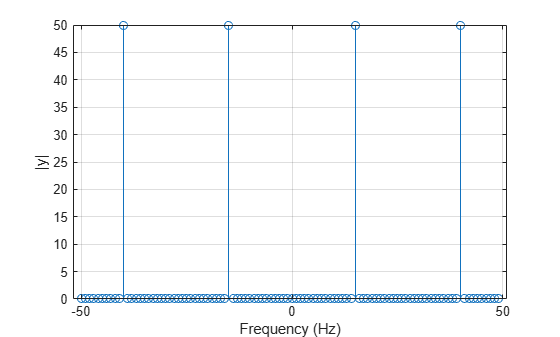# angle

## 语法

theta = angle(z)

## 说明

theta = angle(z) 为复数数组 z 的每个元素返回区间 [-π,π] 中的相位角。theta 中的角度表示为 z = abs(z).*exp(i*theta)。

## 示例

z = 2*exp(i*0.5)
z = 1.7552 + 0.9589i 
r = abs(z)
r = 2 
theta = angle(z)
theta = 0.5000 

fs = 100; t = 0:1/fs:1-1/fs; x = cos(2*pi*15*t - pi/4) - sin(2*pi*40*t);

y = fft(x); z = fftshift(y); ly = length(y); f = (-ly/2:ly/2-1)/ly*fs; stem(f,abs(z)) xlabel 'Frequency (Hz)' ylabel '|y|' gridtol = 1e-6; z(abs(z) < tol) = 0; theta = angle(z); stem(f,theta/pi) xlabel 'Frequency (Hz)' ylabel 'Phase / \pi' grid## 算法

angle 函数采用复数 z = x + iy 并使用 atan2 函数计算 xy 平面上在 x 轴正方向与从原点到点 (x,y) 的射线之间形成的角度。